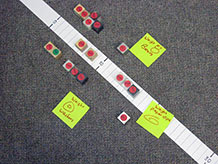Investigating Weight 4:

# How much heavier is one cube than another?

## 3. Make meaning

All Class 15 Mins Notebook

### Purpose of the discussion

The purpose of this discuss is to consolidate learning from this investigation.

Gather students together and show them the plastic weight line. Explain that the numbers on the weight line refer to the number of measuring units that the class decided on, i.e., steel washers, or paper clips, or counting bears. Place an index card adjacent to the line labeled with the chosen unit of measure. Then invite each team to place its cubes in the appropriate place on the line.Check students‘ understanding of the visual array by asking a few questions that they can answer by observation. For example,

Note: This is the first time students will use the weight line. Are they familiar with how it is used? You may want to ask a volunteer to place the first cube on the line while others watch. Point out the weight of that cube in the table and then ask the student to find the same number on the line.

• How many cubes weigh less than 50 paper clips?
• Which two cubes are closest in weight?

Looking at both the data table and the weight line, can students find the same cube in both representations? Ask several volunteers to locate different cubes both in the table and on the line.

### Engage students in the focus question

Our investigation question is:

How much heavier is one cube than another?

What did we do?

• used uniform units of weight to weigh the cubes

Why did we do it?

• we couldn’t compare weights when the units were different so we agreed to use [paper clips, washers, bears] to weigh the cubes

What did we find out?

• refer to the cubes placed on the weight line

How much heavier than the pine cube is the PVC cube?

Which cube is about twice the weight of the PVC cube?

How does each of these representations — the data table and the weight line — help you to answer these questions?

What advantages do you see with each representation?

Refer students to the page in their notebooks, and ask them to answer the four questions.